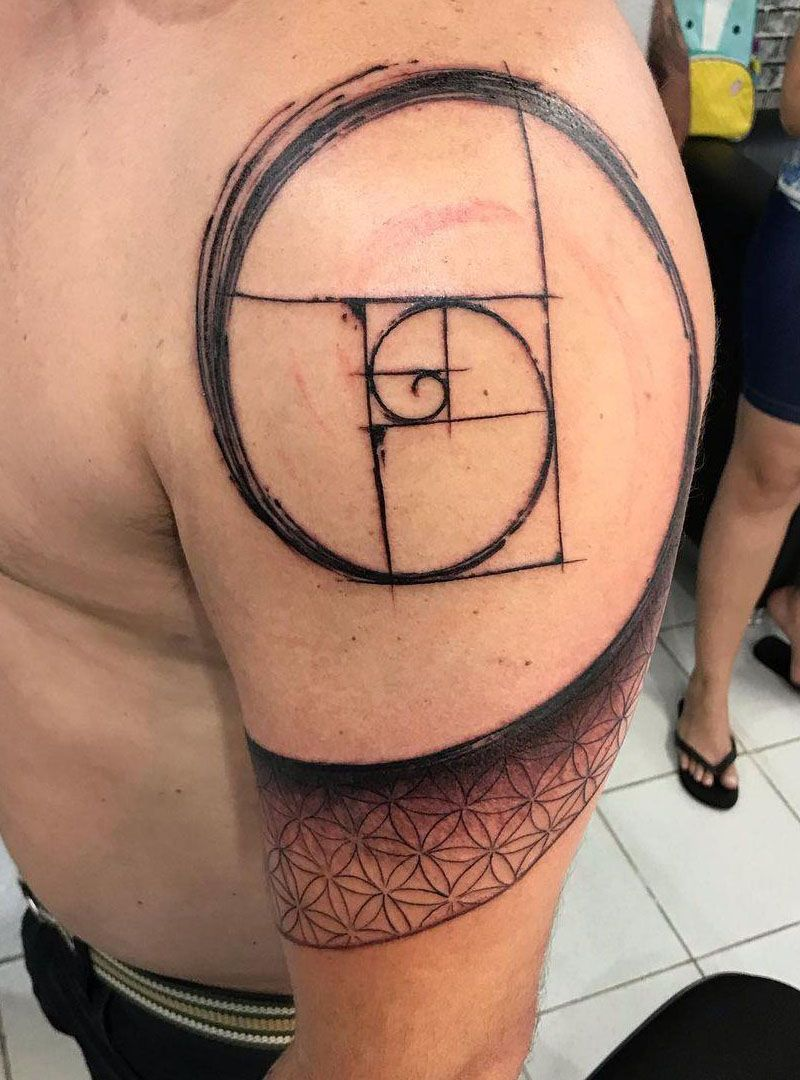# 30 Pretty Fibonacci Tattoos You Will Love

Fibonacci numbers in Fibonacci series often appear in front of our eyes – such as pinecones, pineapples, arrangement of leaves, petals of some flowers (typically Sunflower petals), honeycomb, dragonfly wings, golden rectangle, golden section, equiangular spiral, etc.

Fibonacci spiral, also known as “golden spiral”, is a spiral curve drawn according to Fibonacci sequence. There are many patterns of Fibonacci spiral in nature, which is the most perfect classic golden ratio in nature. The rule of drawing is to draw a 90 degree sector in a rectangle made up of squares with Fibonacci numbers as sides, and the connected arc is the Fibonacci spiral.Source: @aeroquadros